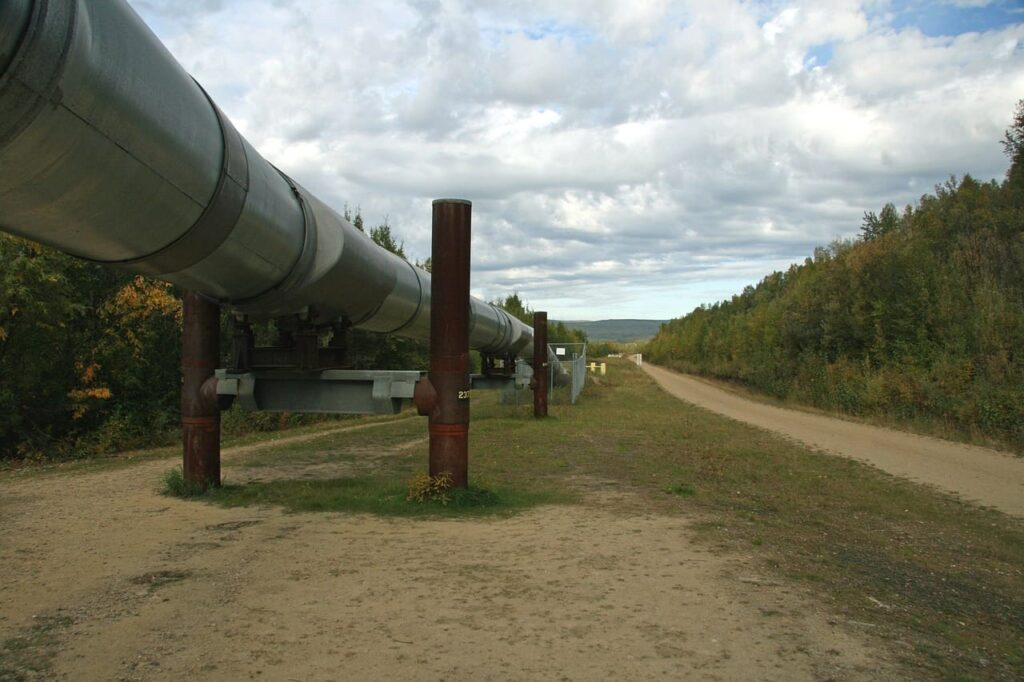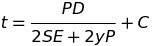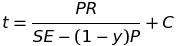# How To: Calculate The Minimum Required Thickness Of Piping

While completing 3rd Class ASME code calculations involving cylindrical components you’ll be required to know how to calculate the minimum required thickness of piping. The objective of this post is to provide you with a clear understanding of how to accomplish this and explain the material covered in:

All page numbers referenced below are from 2007 ASME Boiler & Pressure Vessel Code

## Formula

To calculate the minimum required thickness of piping required for various applications you will use a formula contained within ASME Boiler & Pressure Vessel Code PG-27 Cylindrical Components Under Internal Pressure page 10. Specifically PG-27.2.2 page 10

PG-27.2.2 Piping, Drums, and Headers. (based on strength of weakest course) It is important to remember any cylinder with an outside diameter less then 125mm is to be considered tubing and the equation provided in PG-27.2.1 page 8 must be used.

PG-27.2.2 Formula to calculate the minimum required thickness:

Outside Diameter Equation## Formula Variables

The symbols used within the PG-27 formulas are contained within paragraph PG-27.3 page 10 and are defined as follows:

C = Minimum allowance for threading and structural stability (mm) (PG-27.4, note 3) page 11

D = Or O.D. is Outside diameter of cylinder (mm) “In this case piping”

E = Efficiency of longitudinal welded joints or of ligaments between openings, whichever is lower (the values allowed for E are listed in PG-27.4, note 1) page 11

e = Thickness factor for expanded tube ends (mm) (see PG-27.4, note 4) page 11

P = Maximum allowable working pressure “Gauge Pressure” (MPa) (see PG-21, refers to gauge pressure)

R = Inside radius of cylinder (mm) “In this case piping”

S = Maximum allowable stress value at the operating temperature of the metal (Section II, Part D, Table 1A. See PG-27.4, note 2) page 11. It is important when determining the maximum allowable stress value to check (PG-6 plate materials) page 4 and (PG-9 boiler tube materials) page 5 before starting calculations as this information will determine the correct stress table to use by indicating whether the material is carbon steel or an alloy steel.

t = minimum required thickness (mm) (see PG-27.4, note 7) page 12

y = temperature coefficient (see PG-27.4, note 6) page 11

## How To: Calculate The Minimum Required Thickness Of Piping Questions

Note: All code questions are to be calculated in (mm) and (MPa) unless otherwise stated. Convert accordingly and properly before the calculation.

Question # 5 How To Determine The Minimum Required Thickness Of Piping

Question # 6 How To Determine The Minimum Required Thickness Of Piping

## Summary

Let me know if the examples provided helped you understand how to calculate the minimum required thickness for piping. If you have any questions, feedback, or ideas for the content provided above, let me know in the comment section.

Power Engineering 101